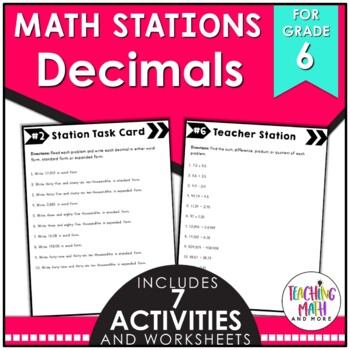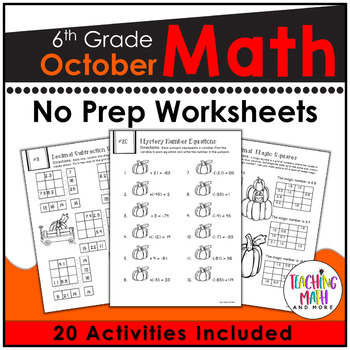## Friday, September 30, 2016

### Teaching Operations with Decimals {Grade 6}

Operations with decimals is an important skill for every student to master. Just think of the multiple ways you use decimals every day! Money, time, food, travelling, measurement and the list goes on. Almost everything that can be measured, can be measured with decimals. That's a lot of decimals! Decimals help us to be precise and exact when measuring. Being able to use operations with decimals correctly is important for everyone to master, especially your building contractor! Here are some fun ways to learn and review operations with decimals:This math station activity is intended to help students understand how to fluently divide multi-digit numbers, and to fluently add, subtract, multiply, and divide multi-digit decimals using the standard algorithm for each operation.
Included are:
-6 different stations to engage students
-Teacher facilitated activity for 60-90 minutes of classroom time
-Stations include:
1. Vocabulary
3. Practice of Decimal Operations
4. Additional Practice of Decimal Operations
5. Real World applications of Decimals
6. Word problems of Decimal Operations
-Student Station Guide helps students record their answersThese interactive notebook activities are intended to help students understand how to fluently divide multi-digit numbers, and to fluently add, subtract, multiply, and divide multi-digit decimals using the standard algorithm for each operation.
Included are:
-4 different Decimals activities to engage students
-Teacher facilitated activity for 60-90 minutes of classroom time
-Activities include: Vocabulary, Practice of writing Decimals, Adding & Subtracting Decimals, and Multiplying & Dividing Decimals.
-Examples of Completed Interactive Math Notebook Activities are included